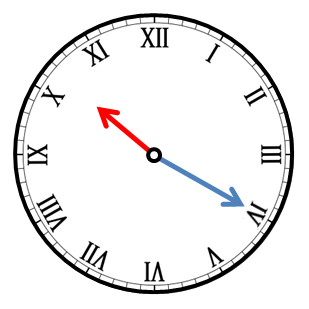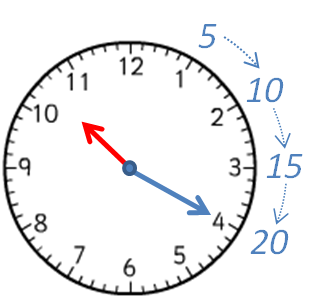• ## Accessibility

Open the accessibility toolbar to change fonts and contrast, access a dictionary, use a ruler and more

Here are two clocks.

One has normal numbers.

The other has Roman Numerals.

Both show the same time.Count the minutes in 5s as you go around until you reach the long blue hand.The long blue hand is pointing to the 4 or IV, which is 4 × 5 = 20 minutes, so the time will be something  20.

The short red hand is pointing between the 10 and the 11 or the X and the XI, so the time is:

10:20

or 20 past 10 because it is 20 minutes past 10 o'clock.

Here are two more clocks, showing the same time.Count the minutes in 5s as you go around until you reach the long blue hand.The long blue hand is pointing to the 7 or the VII, which is 7 × 5 = 35 minutes, so the time will be something  35.

The short red hand is pointing between the 2 and the 3 or the II and the III, so the time is:

2:35

or 25 to 3 because there are 25 mins (minutes) until 3 o'clock.

10 questions